Subjective Information Measure

and Rate Fidelity Theory

Chen-Guang Lu

Independent Researcher[]

email: survival99@hotmail.com

Abstract--With help of fish-covering model, this paper intuitively explains how to extend  Hartley’s information formula to the generalized information formula step by step for  measuring subjective information: metrical information (such as conveyed by thermometers), sensory information (such as conveyed by color vision), and semantic information (such as conveyed by weather forecasts). The pivotal step is to differentiate condition probability and logical condition probability of a message. The paper illustrates the rationality of the formula, discusses the coherence of the generalized information formula and Popper’s knowledge evolution theory. For optimizing data compression, the paper discusses rate-of-limiting-errors and its similarity to complexity-distortion based on Kolmogorov’s complexity theory, and improves the rate-distortion theory into the rate-fidelity theory by replacing Shannon’s distortion with subjective mutual information. It is proved that both the rate-distortion function and the rate-fidelity function are equivalent to a rate-of-limiting-errors function with a group of fuzzy sets as limiting condition, and can been expressed by a formula of generalized mutual information for lossy coding, or by a formula of generalized entropy for lossless coding. By analyzing the rate-fidelity function related to visual discrimination and quantizing bits of pixels of images, the paper concludes that subjective information is less than or equal to objective (Shannon’s) information; there is an optimal matching point at which two kinds of information are equal; the matching information increases with visual discrimination (defined by confusing probability) rising; for given visual discrimination, too high resolution of images or too much objective information is wasteful.

Index Terms--Shannon’s theory, generalized information theory, subjective information, metrical information, sensory information, semantic information, Popper’s theory, complexity-distortion, rate-distortion, rate-fidelity.

# I. Introduction

To measure sensory information and semantic information, I set up a generalized information theory thirteen years ago [4-8] and published a monograph focusing on this theory in 1993 . But, my researches are still rarely known by English researchers of information theory. Recently, I read some papers about complexity distortion theory ,  based on Kolmogorov’s complexity theory. I found that, actually, I had discussed complexity-distortion function and proved that the generalized entropy in my theory was just such a function, and had concluded that the complexity-distortion function with size-unequal fuzzy error-limiting balls could be expressed by a formula of generalized mutual information. I also found that some researchers did some efforts  similar to mine for improving Shannon’s rate-distortion theory.

This paper first explains how to extend Hartley’s information formula to the generalized information formula,  and then discusses the generalized mutual information and some questions related to Popper’s theory, complexity distortion theory, and rate-distortion theory.

# II.  Hartley’ s Information Formula and a Story of Covering Fish

Hartley’s information formula is 

I=logN,                                         (1)

where I denotes the information conveyed by the occurrence of one of  N events with equal probability. If a message y tells that uncertain extension changes from N1  to N2, then information conveyed by y is

Ir=I1-I2=logN1-logN2=log(N1/N2).                (2)

We call (2) relative information formula. Before discussing its properties, I tell a story about covering fish with fish covers.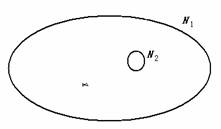Figure 1. Fish-covering model for relative information Ir

Fish covers are made of bamboo. A fish cover looks like a hemisphere with a round hole at top for human hand to catch fish. Fish covers are suitable for catching fish in adlittoral pond. When I was a teenage, after seeing peasants catch fish with fish covers, I found a basket with a hole at bottom and followed those peasants to catch fish. Fortunately, I successfully caught some fish. Then I compared my basket with much bigger fish covers to concluded that the fish cover is bigger so that covering fish is easier; yet, catching fish with hand is more difficult; if a fish cover is big enough to cover the pond, it must be able to cover fish; yet, it is useless because catching fish with hand is the same difficult as before; when one uses the basket or smaller fish cover to cover fish, though covering fish is more difficult, catching fish with hand is much easier.

An uncertain event is alike a fish with random position in a pond. Let a sentence y=” Fish is covered”; y will convey information about the position of fish. Let N1 be the area of the pond, N2 be the area covered by the fish cover, then information conveyed by y is Ir=log( N1/ N2). The smaller  N2  is than N1, the bigger the information amount is. This just reflects the advantage of the basket. If N2= N1then I=0. This just tells us that covering fish is meaningless if the cover is the same big as the pond. As for the advantage of fish covers (with less failure) in comparison with the basket, the above formula cannot tell because in the classical information theory, there seems a hypothesis that the failures of covering fish never happen.

# III. Improving the Fish-covering Formula with Probability

Hartley’s information formula requires N events with equal probability P=1/N. Yet, the probabilities of events are unequal in general. For example, the fish stays in deep water in bigger probability and in shallow water in smaller probability. In these cases, we need to replace 1/N with probability P so that

I=log(1/P)                                           (3)

and

Ir =log(P2/ P1).                                       (4)

# IV. Relative Information Formula with  a Set as Condition

Let X denote the random variable taking values from set A={x1, x2, …} of events, Y denote the random variable taking values from set B={y1, y2,…} of sentences or messages. For each yj, there is a subset Aj  of A and yj =“xi Aj ”, which can be cursorily understood as “Fish xi is in cover Aj ” . Then P1 above becomes P(xi),  P2 becomes P(xi|xi Aj). We simply denote P(xi|xi Aj) by  P(xi|Aj), which is conditional probability with a set as condition. Hence, the above relative information formula becomes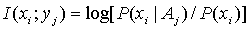.                (5)

For convenience, we call this formula as the fish-covering information formula.

Note that the most important thing is generally P(xi|Aj) P(xi |yj), because

P(xi|yj)= P(xi|“xi Aj”)=P(xi |“xi Aj” is reported);

yet,

P(xi|Aj)= P(xi|xi Aj)=P(xi |“xi Aj” is true),

where yj may be an incorrect reading datum, a wrong message, or a lie, yet, xi Aj means that yj  must be correct. If they are always equal, then formula (5) will become classical information formula,               (6)

whose average is just Shannon mutual information .

# V. Bayesian Formula for the Fish-covering Information Formula

Let the feature function of set Aj   be  Q(Aj|xi) {0,1}. According to Bayesian formula, there is

P(xi |Aj)=Q(Aj | xi )P(xi )/Q(Aj ),                  (7)

where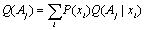. From (5) and (7), we have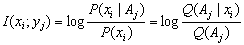,           (8)

which (illustrated by Figure 2) is the transition from classical information formula to generalized information formula.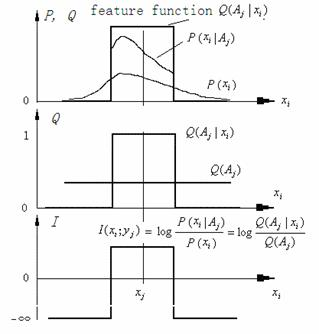Figure 2. Illustration of fish-covering information formula related to Bayesian formula

The reading datum of a thermometer may be considered as reporting sentence yjB={y1, y2,…}and real temperature as the fish position xi A={x1, x2, …}. Let yj =“xi Aj”, and Aj = [xj-x, xj+x] according to the resolution of the thermometer and eyes' visual discrimination. Hence, we can use the fish-covering information formula to measure thermometric information.

# VI. Generalized Information Formula with a Fuzzy Set as Condition

Information conveyed by a reading datum of thermometer and information conveyed by a forecast “The rainfall will be about 10 mm” are the same in essence. Using a clear set as condition as above is not good enough because the information amount should change with xi continuously. We wish that the bigger the error (i.e. xi-xj ), the less the information. Now, using a fuzzy set to replace the clear set as condition, we can realize this purpose (see Figure 4).

Now, we consider yj as sentence “X  is xj ” (or say yj =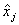).  For a fuzzy set Aj whose feature function Q(Aj|xi) takes value from [0, 1] and Q(Aj|xi) can be considered as confusing probability of xi  with xj. If i=j, then the confusing probability reaches its maximum 1.

Actually, the confusing probability Q(Aj|xi) is only different parlance of the membership grade of xi in fuzzy set Aj or the logical probability of proposition yj (xi). There is

Q(Aj|xi)=feature function of Aj

=confusing probability or similarity of xi with xj

=membership grade of xi in Aj

=logical probability or creditability of proposition yj(xi )

The discrimination of human sense organs, such as visual discrimination for gray levels of pixels of images, can also be described by confusing probability functions. In these cases, a sensation can be considered as a reading datum yj=of the thermometer. The visual discrimination function of xj is Q(Aj|xi), i=1, 2,… where Aj is a fuzzy set containing all xi that are confused with xj. We may use the statistic of random clear sets to obtain this function  (see Figure 3).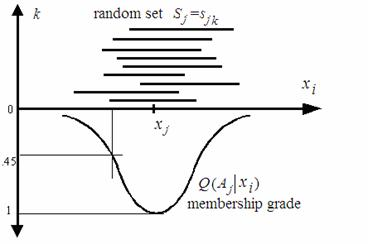Figure 3 Confusing probability function from clear sets

First we do many times experiments to get  the clear sets sjk, k=1, 2, …, n, by putting xj on one side of a screen, xi on another side of the screen. And then we calculate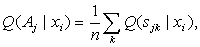(9)

Now, replacing a clear set with a fuzzy set as condition, we get the generalized information formula: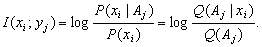(10)

It looks the same as the fish-covering information formula (8), but Q(Aj|xi) [0,1] instead of Q(Aj|xi) {0,1}. And also, this formula allows wrong reading data or messages, bad forecasts, or lies which convey negative information. The generalized information formula can be understood as fish-covering information formula with fuzzy cover. Because of fuzziness, generally, negative information amount is finite. The property of the formula can be illustrated by Figure 4.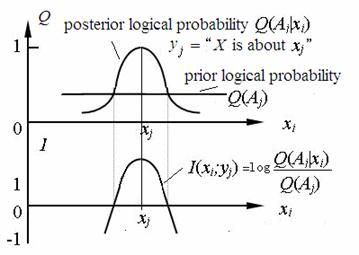Figure 4 Generalized information formula for measuring metrical information, sensory information, and number-forecasting information

Figure 4 tells us that when a reading datum or a sensation yj=is provide, the bigger the difference of xi from xj, the less the information; and the less the Q(Aj), the bigger the absolute value of information. From this formula, we can conclude that information amount is also not only depends on the correctness of reflection, but also depends on the precision of reflection.

# VII. Coherence of the Semantic Information Measure and Popper’s Criterion of Advance of Knowledge

The generalized information formula can also be used to measure semantic information in general, such as information from weather forecast “Tomorrow will be rainy or heavy rainy”.  We may assume that for any proposition yj, there is a Plato’s idea xj which makes Q(Aj|xj)=1. The idea xj is probably not in Aj. Hence, any logical condition probability  Q(Aj|xi) can be considered as the confusing probability of xi with the idea xj.

From my view-point, forecasting information is more general information in comparison with descriptive information. If a forecast is always correct, then the forecasting information will become descriptive information.

About the criterion of advance of scientific theory, philosopher Karl Popper wrote:

“The criterion of relative potential satisfactoriness… characterizes as preferable the theory which tell us more; that is to say, the theory which contains the greater amount of empirical information or content; which is logically strong; which has the greater explanatory and predictive power; and which can therefore be more severely tested by comparing predicted facts with observations. In short, we prefer an interesting, daring, and highly informative theory to a trivial one.” ( in , pp. 250)

Clearly, Popper used information as the criterion to value the advance of scientific theories. According to Popper’s theory, the more easily a proposition is falsified logically and the more it can go through facts (in my words, the less the prior logical probability Q(Aj) is, and the bigger the posterior logical probability Q(Aj|xi) is ), the more information it conveys and the more meaningful it is. Contrarily, a proposition that can not be falsified logically (in my words, Q(Aj|xi)Q(Aj)1) conveys no information and is insignificant in science. Obviously, the generalized information measure is very coherent with Popper’s information criterion; the generalized information formula functions as a bride between Shannon’s information theory and Popper’s knowledge evolution theory.

# VIII．Generalized Fullback’s Information and Generalized Mutual Information

Calculating the average of I(xi; yj) in (10), we have generalized Fullback’s information formula for given yj:.          (11)

Actually, the probabilities on right of log should be prior probabilities or logical probabilities, the probability on left of log should be posterior probability. Since now we differentiate two kinds of probabilities and use Q(.) for those  probabilities after log. Hence the above formula becomes.            (12)

We can prove that as Q(X|Aj)= P(X|Aj), which means subjective probability forecasts conforms to objective statistic, I(X; yj) reaches its maximum. The more different the Q(X) is from P(X|Aj), which means that the facts are more unexpected, the bigger the I(X; yj) is. This formula also conforms to Popper’s theory.

Further, we have generalized mutual information formula(13)

where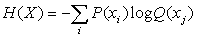(14)(15)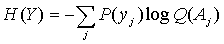(16)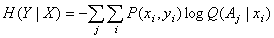(17)

I call H(X) forecasting entropy, which reflects the average coding length when we economically encode X according to Q(X) while real source is P(X), and reaches its minimum as Q(X)= P(X).  I call  H(X|Y) posterior forecasting entropy, call H(Y)  generalized entropy, and call H(Y|X)  generalized condition entropy or fuzzy entropy .

I think that the generalized information is subjective information and Shannon information is objective information. If two weather forecasters always provide opposite forecasts and one is always correct and another is always incorrect. They convey the same objective information, but the different subjective information. If Q(X)= P(X)  and Q(X|Aj)= P(X|yj) for each j, which means subjective forecasts conform to objective facts, then the subjective mutual information equals objective mutual information.

# IX.  Rate-of-limiting-errors and Its Relation to Complexity-distortion

In , I defined rate-of-limiting-errors, which is similar to complexity distortion . The difference is that the error-limiting condition for rate-of-limiting-errors is a group of sets or fuzzy sets AJ= { A1,  A2…} instead of a group of balls with the same size and clear boundaries for complexity distortion.

We know that the color space of digital images is visually ununiform and human eyes’ discrimination is fuzzy. So, in some cases, such as coding for digital images, using size-unequal balls or fuzzy balls as limiting condition will be more reasonable.

Assume P(Y) is a source; encode Y into X; allow yj is encoded into any xj in  clear set Aj,  j=1, 2…; then the minimum of Shannon mutual information for different P(X|Y) is defined as  rate-of-limiting-errors R(AJ).

I had proved that R(AJ)= H(Y). To realize this rate, there must be P(X|yj)= Q(X|Aj) for each j .

Furthermore, when the limiting sets are fuzzy, i.e. P(X|yj) ≤ Q(X|Aj) for each j as Q(Aj|xi)<1, there is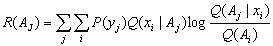(18)

To realize this rate, there must be  P(X)= Q(X) and P(X|yj)= Q(X|Aj) for each j so that Shannon’s mutual information equals the generalized mutual information.

Now, from the view-point of the complexity distortion theory, the generalized entropy H(Y) is just prior complexity, the fuzzy entropy H(Y|X) is just the posterior complexity, and I(X;Y) is the reduced complexity.

# X. Rate Fidelity Theory:  Reformed Rate Distortion Theory

Actually, Shannon ever mentioned fidelity criterion for lossy coding. He used the distortion as the criterion for optimizing lossy coding because the fidelity criterion is hard to be formulated.  However, distortion is not a good criterion in most cases.

How do we value a person? We value him according to not only his errors but also his contributions. For this reason, I replace the error function dij=d(xi, yj) with generalized information Iij= I(xi; yj) and distortion d(X, Y) with generalized mutual information I(X; Y) as criterion to search the minimum of Shannon mutual information Is(X; Y) for given P(X)=Q(X) and the lower limit G of I(X; Y).  I call this criterion  the fidelity criterion, call the minimum the rate-fidelity function R(G), and call the reformed theory the rate fidelity theory.

In a way similar to that in the classical information theory , we can obtain the expression of function R(G) with parameter s:(19)

where s=dR/dG indicates the slope of function R(G) ( see Figure 5) and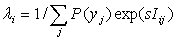.

We define a group of sets BI= {B1,  B2…}, where  B1,  B2… are subset of  B={y1, y2,…}, by  fuzzy feature function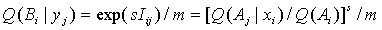(20)

where m is the maximum of exp(sIij); then from (19) and (20) we have(21)

This function is just the rate-of-limiting-errors with a group of fuzzy sets BI={B1,  B2…} as limiting condition while coding X in A into Y in B. From this formula, we can find there is profound relationship between rate-of-limiting-errors and rate-fidelity (or rate-distortion). In the above formulas, if we replace Iij with dij=d(xi, yj), (21) is also tenable. So, actually rate-distortion function can be expressed by a formula of generalized mutual information.

In , I defined information value V by the increment of growing speed of fund  because of information, and suggested to use the information value as criterion to optimize communication in some cases to get function rate-value R(V), which is also meaningful.

# XI. Rate-fidelity Function for Optimizing Image Communication

For simplicity, we consider how subjectively visual information is related to visual discrimination and quantizing grades of gray levels of pixels of images,.

Let the gray level of quantized pixel be a source and the gray level is xi=i, i=0, 1... b =2k -1 with normal probability distribution whose expectation=b/2 and standard deviation= b/8 (see  for details). Assume that after decoding the pixel also has gray level yj=j=0, 1... b; the perception caused by yj is also denoted by yj; and discrimination function or confusing probability function  of xj  is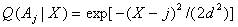(22)

where d is discrimination parameter. The smaller the d, the higher the discrimination.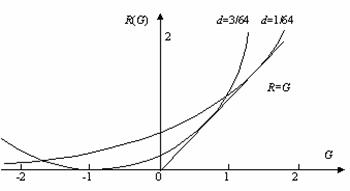Figure 5 Relationship between d and R(G) for b=63

Figure 5 indicates that when R=0, G<0, which means that if a coded image has nothing to do with the original image, we still believe it reflects the original image, then the information will be negative. When G=-2, R>0, which means that certain objective information is necessary when one uses lies to deceive enemy to some extent; or say, lies based on facts are more terrible than lies based on nothing. The each line of function R(G) is tangent with the line R=G, which means there is a matching point at which objective information is equal to subjective information, and the higher the discrimination (the less the d), the bigger the matching information amount. The slope of R(G) becomes bigger and bigger with G increasing, which tell us that for given discrimination, it is limited to increase subjective information.

Figure 6 tells us that for given discrimination, there exists the optimal quantizing-bit k' so that the matching value of G and R reaches the maximum. If k<k', the matching information increases with k; if k>k', the matching information no longer increases with k. This means that too high resolution of images is unnecessary or uneconomical for given visual discrimination.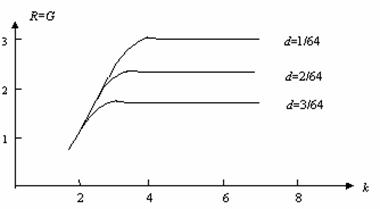Figure 6  Relationship between matching value of R with G, discrimination parameter d, and quantizing bit k

# References

  T. Berger, Rate Distortion Theory, Englewood Cliffs, N.J.: Prentice-Hall, 1971.

   M. S. Daby and E. Alexandros, “Complexity Distortion Theory”, IEEE Tran. On Information Theory, Vol. 49, No. 3, 604-609, 2003.

  R. V. L. Hartley, “Transmission of information”, Bell System Technical Journal, 7 , 535, 1928.

  C.-G. Lu, “Coherence between the generalized mutual information formula and Popper's theory of scientific evolution”(in Chinese), J. of Changsha University, No.2, 41-46, 1991.

 C.-G. Lu, A Generalized Information Theory (in Chinese), China Science and Technology University Press, 1993

  C.-G. Lu, “Coding meaning of generalized entropy and generalized mutual information” (in Chinese), J. of China Institute of Communications, Vol.15, No.6, 38-44, 1995.

  C.-G. Lu, Portfolio’s Entropy Theory and Information Value, (in Chinese), China Science and Technology University Press, 1997

  C.-G. Lu, “A generalization of Shannon's information theory”, Int. J. of General Systems, Vol. 28, No.6, 453-490, 1999.

   G. Peter and P. Vitanyi, Shannon information and Kolmogorov complexity,  IEEE Tran. On Information Theory,  submitted,  http://homepages.cwi.nl/~paulv/papers/info.pdf

 K. Popper, Conjectures and Refutations―the Groth of Scientific Knowledge, Routledge, London and New York, 2002.

 C. E. Shannon, “A mathematical theory of communication”, Bell System Technical Journal, Vol. 27, pt. I, pp. 379-429; pt. II, pp. 623-656, 1948.

 P. Z. Wang, Fuzzy Sets and Random Sets Shadow (in Chinese), Beijing Normal University Press, 1985.

[] Author’s personal website: http://survivor99.com/lcg/english